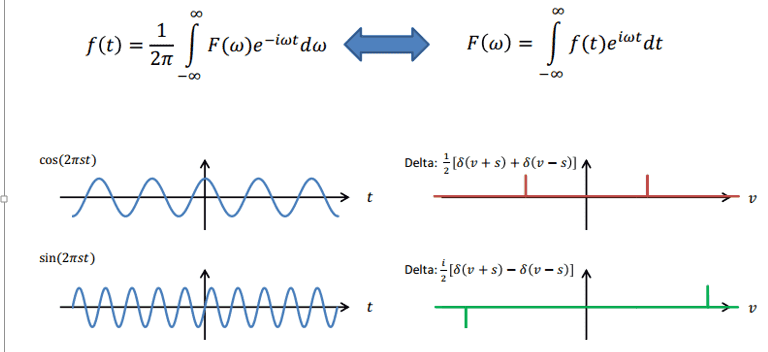# Fourier Transform of a sin(2pi*x)

I have been very briefly introduced to Fourier transformations but the topic was not explained especially well (or I just didn't understand it!)

We were shown the graphs with equations below and then their fourier transformation (RHS). I understand the one for cos(2pist) but NOT the sin(2pist).

Here is my reasoning: The sin wave shown has a constant frequency (that is larger than the cosine function; hence its frequency values are larger). It is possible to have a "-ve" frequency because waves can travel in both directions. I do not understand why the amplitude on the sin graph is negative though? If it was negative on both it would make sense but it isn't!
I know that both FT boil down to a cosine expression (as the sin expression is lost when integrating between infinities) and that makes it even more confusing for me! Please can someone help me understand. thanksblue_leaf77
Homework Helper
I do not understand why the amplitude on the sin graph is negative though?
Just a wording correction though, amplitude by definition is a positive quantity. As for your question, two functions connected through Fourier transform are in general complex, that is, they have modulus and phase. The reason why the negative frequency component in the FT of sine points down is that because this component are 180 degree out of phase from the positive frequency component.
I know that both FT boil down to a cosine expression (as the sin expression is lost when integrating between infinities) and that makes it even more confusing for me!
You don't integrate the delta function alone, do you. There is also ##e^{i\omega t}## in the integrand.

Just a wording correction though, amplitude by definition is a positive quantity. As for your question, two functions connected through Fourier transform are in general complex, that is, they have modulus and phase. The reason why the negative frequency component in the FT of sine points down is that because this component are 180 degree out of phase from the positive frequency component.

But surely the cos points are also 180 degrees out of phase? I am not too sure I understand what you mean by this

Oh Wait. Do you mean because sin is an odd function whereas cos is an even function?

blue_leaf77
Homework Helper
But surely the cos points are also 180 degrees out of phase? I am not too sure I understand what you mean by this
No, the Fourier transform of cosine is real.
A complex number ##z## can be expresed as ##z=|z|e^{i\theta}## where ##|z|## the modulus and ##\theta## the phase. You can write the Fourier transform of sine as
$$\frac{1}{2}(\delta(\nu+s) - \delta(\nu-s)) = \frac{1}{2}(\delta(\nu+s) + e^{i\pi} \delta(\nu-s))$$
EDIT: the Fourier transform of cosine is real and symmetric.

Last edited:
No, the Fourier transform of cosine is real.
A complex number ##z## can be expresed as ##z=|z|e^{i\theta}## where ##|z|## the modulus and ##\theta## the phase. You can write the Fourier transform of sine as
$$\frac{1}{2}(\delta(\nu+s) - \delta(\nu-s)) = \frac{1}{2}(\delta(\nu+s) + e^{i\pi} \delta(\nu-s))$$

so are you saying that the "negative" ampltidue on the sin curve reflects the fact that it is the imaginary component of the sin expression (as per Euler formula)

blue_leaf77
Homework Helper
it is the imaginary component of the sin expression
No, the negative sign appears because of the real component of ##e^{i\pi}## instead.
$$e^{i\pi}=-1$$

•BobP
No, the negative sign appears because of the real component of ##e^{i\pi}## instead.
$$e^{i\pi}=-1$$
I see...thanks for the help.
Truth be told, I understood none of the maths initially but your help has pointed me to videos / math books that have explained the issue to me so I now understand it (I hope!)

Really grateful!